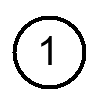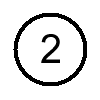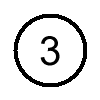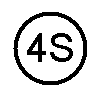There are four die varieties for this coin consisting of four obverse dies and one reverse die.  The differences can be noted in the date.

 1870Penny Obverse Reverse• Obverse 1:
• The spacing between the eight and the seven is 0.81 mm.
• The seven and the zero are out of alignment.
• Digits have been repunched.
• Obverse 2:
• The spacing between the eight and the seven is 0.91 mm.
• The seven and the zero are slightly out of alignment.
• Obverse 3:
• The spacing between the eight and the seven is 0.79 mm.
• The seven and the zero are in alignment.
• Obverse 4S:
• The spacing between the eight and the seven is 0.74 mm.
• The seven and the zero are slightly out of alignment.
• Striations are visible.

• Reverse A:
• There is only one die.The spacing is measured between the digits.  The alignment is measured with respect to the top of the seven.

Obverse Dies:  The DateLarge Spacing between the 8 and the 7
The 7 and 0 are out of alignmentLarge Spacing between the 8 and the 7
The 7 and 0 are in alignmentSmall Spacing between the 8 and the 7
The 7 and 0 are in alignmentSmall Spacing between the 8 and the 7
The 7 and 0 are out of alignment